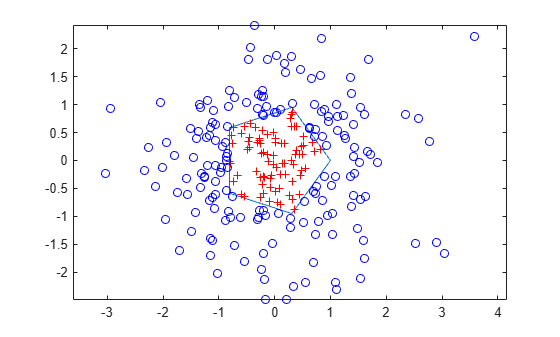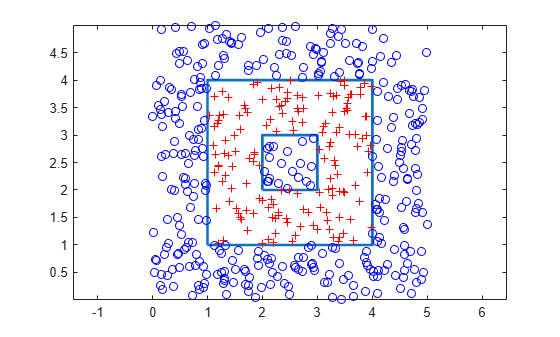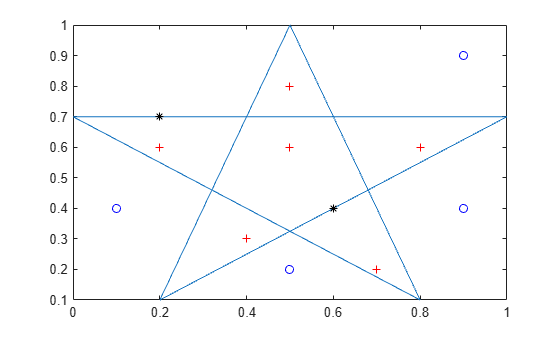# inpolygon

Points located inside or on edge of polygonal region

## Syntax

``in = inpolygon(xq,yq,xv,yv)``
``````[in,on] = inpolygon(xq,yq,xv,yv)``````

## Description

example

````in = inpolygon(xq,yq,xv,yv)` returns `in` indicating if the query points specified by `xq` and `yq` are inside or on the edge of the polygon area defined by `xv` and `yv`.```

example

``````[in,on] = inpolygon(xq,yq,xv,yv)``` also returns `on` indicating if the query points are on the edge of the polygon area.```

## Examples

collapse all

Define a pentagon and a set of points. Then, determine which points lie inside (or on the edge) of the pentagon.

Define the x and y coordinates of polygon vertices to create a pentagon.

```L = linspace(0,2*pi,6); xv = cos(L)'; yv = sin(L)';```

Define x and y coordinates of 250 random query points. Initialize the random-number generator to make the output of `randn` repeatable.

```rng default xq = randn(250,1); yq = randn(250,1);```

Determine whether each point lies inside or on the edge of the polygon area. Also determine whether any of the points lie on the edge of the polygon area.

`[in,on] = inpolygon(xq,yq,xv,yv);`

Determine the number of points lying inside or on the edge of the polygon area.

`numel(xq(in))`
```ans = 80 ```

Determine the number of points lying on the edge of the polygon area.

`numel(xq(on))`
```ans = 0 ```

Since there are no points lying on the edge of the polygon area, all 80 points identified by `xq(in)`, `yq(in)` are strictly inside the polygon area.

Determine the number of points lying outside the polygon area (not inside or on the edge).

`numel(xq(~in))`
```ans = 170 ```

Plot the polygon and the query points. Display the points inside the polygon with a red plus. Display the points outside the polygon with a blue circle.

```figure plot(xv,yv) % polygon axis equal hold on plot(xq(in),yq(in),'r+') % points inside plot(xq(~in),yq(~in),'bo') % points outside hold off```Find the points inside a square with a square hole.

Define a square region with a square hole. Specify the vertices of the outer loop in a counterclockwise direction, and specify the vertices for the inner loop in a clockwise direction. Use `NaN` to separate the coordinates for the outer and inner loops.

```xv = [1 4 4 1 1 NaN 2 2 3 3 2]; yv = [1 1 4 4 1 NaN 2 3 3 2 2];```

Define x and y coordinates of 500 random points. Initialize the random-number generator to make the output of `randn` repeatable.

```rng default xq = rand(500,1)*5; yq = rand(500,1)*5;```

Determine whether each point lies inside or on the edge of the polygon area.

`in = inpolygon(xq,yq,xv,yv);`

Plot the polygon and the query points. Display the points inside the polygon with a red plus. Display the points outside the polygon with a blue circle.

```figure plot(xv,yv,'LineWidth',2) % polygon axis equal hold on plot(xq(in),yq(in),'r+') % points inside plot(xq(~in),yq(~in),'bo') % points outside hold off```Query points in the square hole are outside the polygon.

Define the x and y coordinates for a pentagram.

```xv = [0.5;0.2;1.0;0;0.8;0.5]; yv = [1.0;0.1;0.7;0.7;0.1;1];```

Define the x and y coordinates of 12 query points.

```xq = [0.1;0.5;0.9;0.2;0.4;0.5;0.5;0.9;0.6;0.8;0.7;0.2]; yq = [0.4;0.6;0.9;0.7;0.3;0.8;0.2;0.4;0.4;0.6;0.2;0.6];```

Determine whether each point lies inside or on the edge of the polygon area. Also determine whether any of the points lie on the edge of the polygon area.

`[in,on] = inpolygon(xq,yq,xv,yv);`

Determine the number of points lying inside or on the edge of the polygon area.

`numel(xq(in))`
```ans = 8 ```

Determine the number of points lying on the edge of the polygon area.

`numel(xq(on))`
```ans = 2 ```

Determine the number of points lying outside the polygon area (not inside or on the edge).

`numel(xq(~in))`
```ans = 4 ```

Plot the polygon and the points. Display the points strictly inside the polygon with a red plus. Display the points on the edge with a black asterisk. Display the points outside the polygon with a blue circle.

```figure plot(xv,yv) % polygon hold on plot(xq(in&~on),yq(in&~on),'r+') % points strictly inside plot(xq(on),yq(on),'k*') % points on edge plot(xq(~in),yq(~in),'bo') % points outside hold off```Six points lie inside the polygon. Two points lie on the edge of the polygon. Four points lie outside the polygon.

## Input Arguments

collapse all

x-coordinates of query points, specified as a scalar, vector, matrix, or multidimensional array.

The size of `xq` must match the size of `yq`.

Data Types: `double` | `single`

y-coordinates of query points, specified as a scalar, vector, matrix, or multidimensional array.

The size of `yq` must match the size of `xq`.

Data Types: `double` | `single`

x-coordinates of polygon vertices, specified as a vector.

The size of `xv` must match the size of `yv`.

To specify vertices of multiply connected or disjoint polygons, separate the coordinates for distinct loops with `NaN`. Additionally for multiply connected polygons, you must orient the vertices for external and internal loops in opposite directions.

The polygon cannot be self-intersecting and multiply connected due to the ambiguity associated with self-intersections and loop orientations.

Data Types: `double` | `single`

y-coordinates of polygon vertices, specified as a vector.

The size of `yv` must match the size of `xv`.

To specify vertices of multiply connected or disjoint polygons, separate the coordinates for distinct loops with `NaN`. Additionally for multiply connected polygons, you must orient the vertices for external and internal loops in opposite directions.

The polygon cannot be self-intersecting and multiply connected due to the ambiguity associated with self-intersections and loop orientations.

Data Types: `double` | `single`

## Output Arguments

collapse all

Indicator for the points inside or on the edge of the polygon area, returned as a logical array. `in` is the same size as `xq` and `yq`.

• A logical `1` (`true`) indicates that the corresponding query point is inside the polygonal region or on the edge of the polygon boundary.

• A logical `0` (`false`) indicates that the corresponding query point is outside the polygonal region.

Therefore, you can use `in` to index into `xq` and `yq` to identify query points of interest.

 `xq(in)`, `yq(in)` Query points inside or on the edge of the polygon area `xq(~in)`, `yq(~in)` Query points outside the polygonal region

Indicator for the points on the edge of the polygon area, returned as a logical array. `on` is the same size as `xq` and `yq`.

• A logical `1` (`true`) indicates that the corresponding query point is on the polygon boundary.

• A logical `0` (`false`) indicates that the corresponding query point is inside or outside the polygon boundary.

Therefore, you can use `on` and `in` to index into `xq` and `yq` identify query points of interest.

 `xq(on)`, `yq(on)` Query points on the polygon boundary `xq(~on)`, `yq(~on)` Query points inside or outside the polygon boundary `xq(in&~on)`, `yq(in&~on)` Query points strictly inside the polygonal region

## Version History

Introduced before R2006a